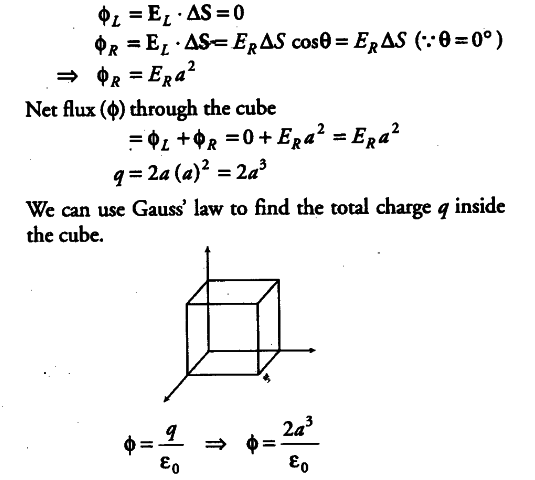# Given the electric field in the region E= 2xi, find the net electric flux through the cube and the charge enclosed by it

Given the electric field in the region E= 2xi, find the net electric flux through the cube and the charge enclosed by it.Since, the electric field has only x-component, for faces normal to X- direction, the angle between E It
and ∆S is ± \pi/2. Therefore, the flux is separately zero
for each of the cube except the shaded ones. The magnitude of the electric field at the left face is
E_{ L } = 0
The magnitude of the electric field at the right face is
E_{ R} =3a

The corresponding fluxes are